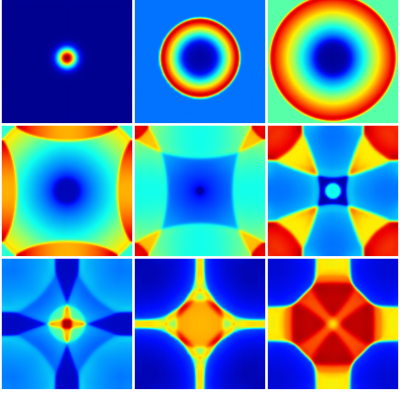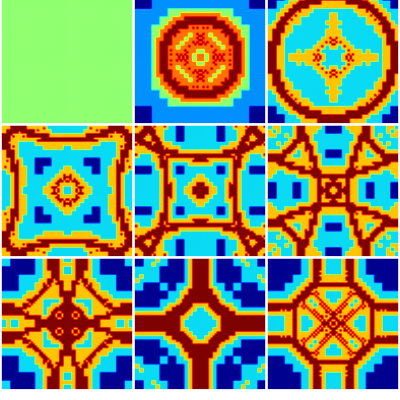# Bouncing Saint-Venant bump

This test case is identical to bump2D.c but using the generic solver for systems of conservation laws rather than the Saint-Venant solver.

#include "conservation.h"

The only conserved scalar is the water depth h and the only conserved vector is the flow rate q.

scalar h[];
vector q[];
scalar * scalars = {h};
vector * vectors = {q};

Using these notations, the Saint-Venant system of conservation laws (assuming a flat topography) can be written \displaystyle \partial_t\left(\begin{array}{c} h\\ q_x\\ q_y\\ \end{array}\right) + \nabla\cdot\left(\begin{array}{c} q_x & q_y\\ \frac{q_x^2}{h} + \frac{Gh^2}{2} & \frac{q_xq_y}{h}\\ \frac{q_yq_x}{h} & \frac{q_y^2}{h} + \frac{Gh^2}{2} \end{array}\right) = 0 with G the acceleration of gravity.

This system is entirely defined by the flux() function called by the generic solver for conservation laws. The parameter passed to the function is the array s which contains the state variables for each conserved field, in the order of their definition above (i.e. scalars then vectors). In the function below, we first recover each value (h, qx and qy) and then compute the corresponding fluxes (f, f and f). The minimum and maximum eigenvalues for the Saint-Venant system are the characteristic speeds u \pm \sqrt(Gh).

double G = 1.;

void flux (const double * s, double * f, double e)
{
double h = s, qx = s, u = qx/h, qy = s;
f = qx;
f = qx*u + G*h*h/2.;
f = qy*u;
// min/max eigenvalues
double c = sqrt(G*h);
e = u - c; // min
e = u + c; // max
}

The solver is now fully defined and we proceed with initial conditions etc… as when using the standard Saint-Venant solver (see bump2D.c for details).

#define LEVEL 7

int main()
{
origin (-0.5, -0.5);
init_grid (1 << LEVEL);
run();
}

event init (i = 0)
{
theta = 1.3; // tune limiting from the default minmod
double b = 200.;
foreach()
h[] = 0.1 + exp(- b*(x*x + y*y));
}

event logfile (i++) {
stats s = statsf (h);
fprintf (stderr, "%g %d %g %g %.8f\n", t, i, s.min, s.max, s.sum);
}

event outputfile (t <= 2.5; t += 2.5/8) {
static int nf = 0;
printf ("file: eta-%d\n", nf);
output_field ({h}, stdout, N, linear = true);

scalar l[];
foreach()
l[] = level;
printf ("file: level-%d\n", nf++);
output_field ({l}, stdout, N);

/* check symmetry */
foreach() {
double h0 = h[];
point = locate (-x, -y);
//    printf ("%g %g %g %g %g\n", x, y, h0, h[], h0 - h[]);
assert (fabs(h0 - h[]) < 1e-12);
point = locate (-x, y);
assert (fabs(h0 - h[]) < 1e-12);
point = locate (x, -y);
assert (fabs(h0 - h[]) < 1e-12);
}
}

#if TREE
astats s = adapt_wavelet ({h}, (double[]){1e-3}, LEVEL);
fprintf (stderr, "# refined %d cells, coarsened %d cells\n", s.nf, s.nc);
}
#endif

## Results

The results are comparable to that of bump2D.c. They are not identical mainly because the standard Saint-Venant solver applies slope-limiting to velocity rather than flow rate in the present case.Evolution of water depth with time.Evolution of level of refinement with time.